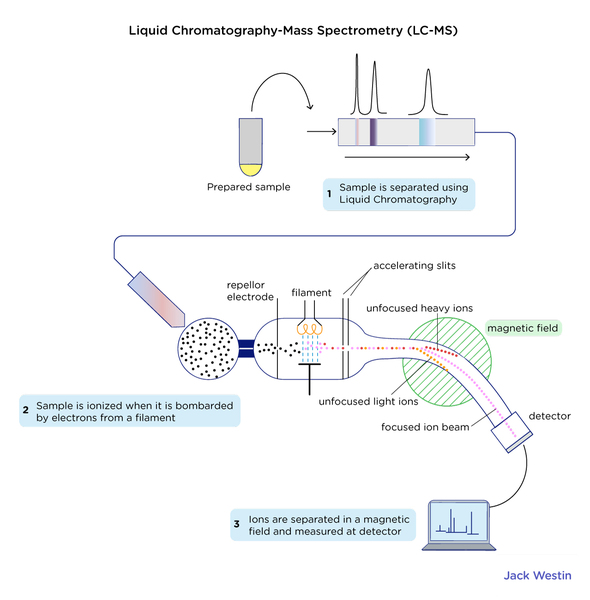MCAT Content / Atomic Nucleus / Mass Spectrometer

### Mass spectrometer

Topic: Atomic Nucleus

Mass Spectrometer is a device to measure mass.

Mass spectrometers separate compounds based on a property known as the mass-to-charge ratio: the mass of the atom divided by its charge.

First, the sample is vapourised into a gaseous state. The gaseous samples are then ionized. Ionization is the process of converting an atom or molecule into an ion by adding or removing charged particles such as electrons or ions. Once the sample is ionized, it is passed through an electric or magnetic field where it accelerates and deflects and is separated out based on its mass. The charged particles are detected at the end by Faraday collectors and the relative current generated by these ions will indicate its relative abundance.

A particle’s mass can be calculated based on parameters such as how long it takes to travel a certain distance or its angle of travel.

Mass spectrometers are so accurate that they can determine the types of elements in a compound or measure the differences between the mass of different isotopes of the same atom.Practice Questions

MCAT Official Prep (AAMC)

Section Bank C/P Section Passage 1 Question 3

Key Points

• Mass spectrometers work on samples in a gaseous state.

• An ion source ionizes the gaseous samples.

• Mass analyzers separate ionized samples according to their mass-to-charge ratio.

• A particle’s mass can be calculated very accurately based on parameters such as how long it takes to travel a certain distance or its angle of travel.

• Mass spectrometers are so accurate that they can determine the types of elements in a compound or measure the differences between the mass of different isotopes of the same atom.

Key Terms

ionization: any process that leads to the dissociation of a neutral atom or molecule into charged particles (ions).

mass-to-charge ratio: the best way to separate ions in a mass spectrometer. This number is calculated by dividing the weight of the ion by its charge.

mass spectrometers: an analytical technique that measures the mass-to-charge ratio of ions. The results are typically presented as a mass spectrum, a plot of intensity as a function of the mass-to-charge ratio

Faraday collectors:  is a metal (conductive) cup designed to catch charged particles in a vacuum

Billing Information